#Function Repository Resource:

# CosDegree

Compute the cosine of an angle given in degrees

Contributed by: Paco Jain (Wolfram Research)
 ResourceFunction["CosDegree"][x] computes the cosine of an angle x, measured in degrees

## Details and Options

Mathematical function, suitable for both symbolic and numerical manipulation.
For certain special arguments, CosDegree automatically evaluates to exact values.
CosDegree can be evaluated to arbitrary numerical precision.
CosDegree automatically threads over lists.

## Examples

### Basic Examples

Compute the cosine of an angle:

 In:=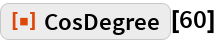Out=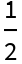Plot over a given range of angles:

 In:=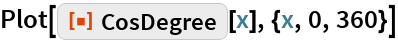Out=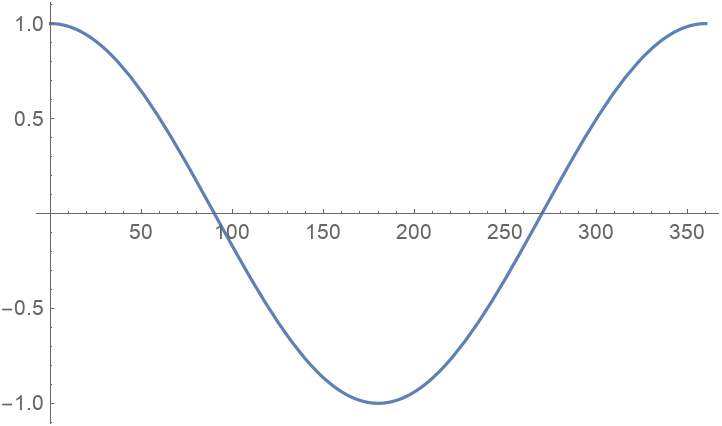### Scope

Evaluate numerically:

 In:=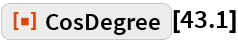Out=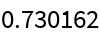Evaluate to high precision:

 In:=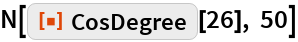Out=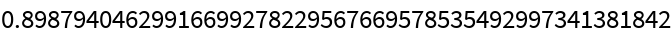### Applications

Draw a circle:

 In:=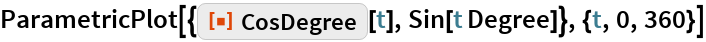Out=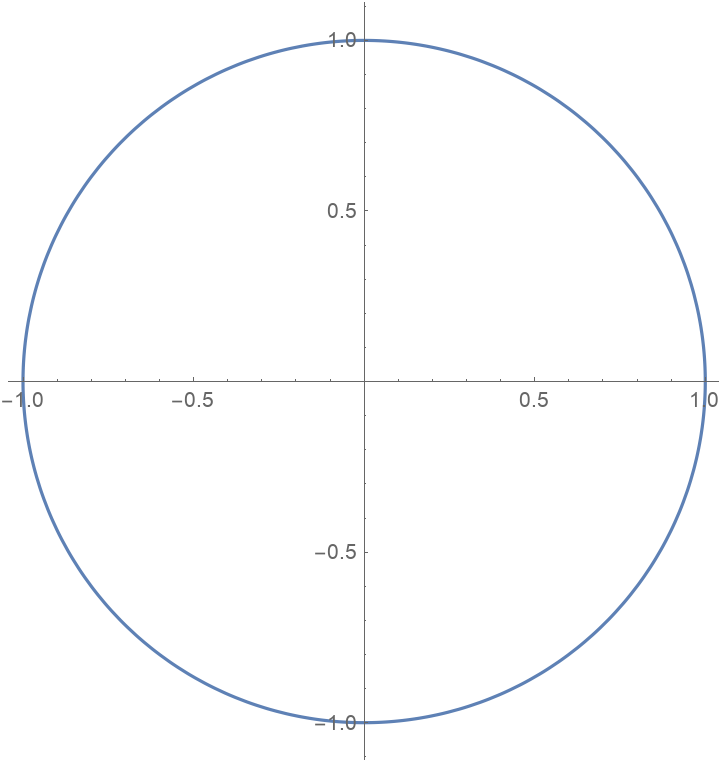## Requirements

Wolfram Language 11.3 (March 2018) or above